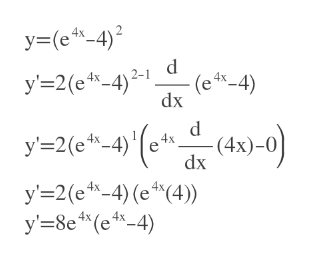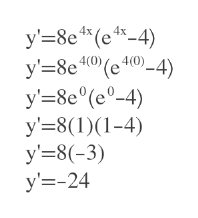Find an equation of the tangent line to the graph of the function at the given point.y = (e4x − 4)2,    (0, 9)y =

Question

Find an equation of the tangent line to the graph of the function at the given point.

y = (e4x − 4)2,    (0, 9)
y =
Step 1

We first have to find y'

We apply exponent rule and chain rule.

y'= 8(e^(4x))*(e^(4x)) -4)help_outlineImage Transcriptionclosey (c44) 2 y'-2(e -4)2-1 -(e 4-4) dx (et(4x)-0) y'-2(e -4) y'2(e -4) (e (4) y' 8e*(e 4-4) fullscreen
Step 2

At x=0 , we calculate y'

That will be the slope ...help_outlineImage TranscriptioncloseУ-38e " (e*-4) 4(0) У'—8e у-8e (е°-4) у-38(1)(1-4) у38(-3) у3-24 fullscreen

Want to see the full answer?

See Solution

Want to see this answer and more?

Our solutions are written by experts, many with advanced degrees, and available 24/7

See Solution
Tagged in

Calculus